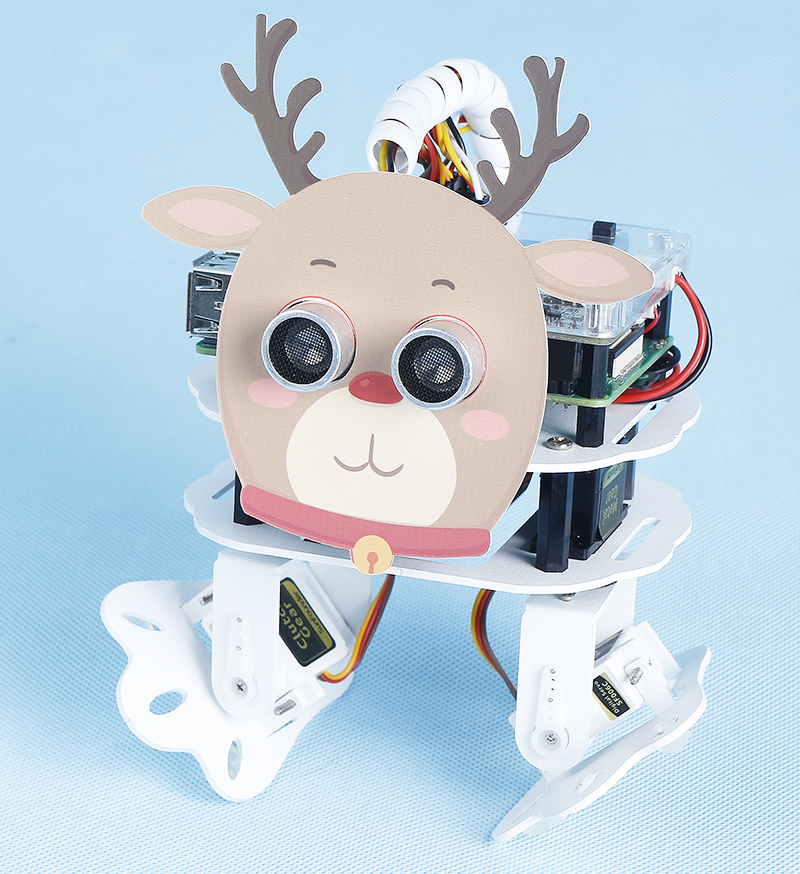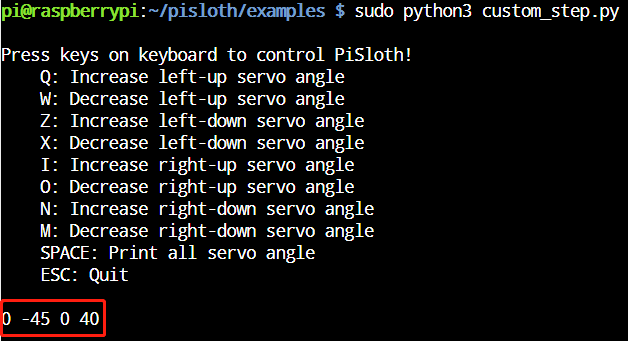# Custom Step¶

In the previous projects, we used a lot of actions that we wrote, so how are these actions composed and done? Generally speaking, an action is composed of one or more steps.

In this project, we will learn how to customize PiSloth’s step.

Note

Run the Code

```cd /home/pi/pisloth/examples
sudo python3 custom_step.py
```

Once the code has been run, press the following keys to adjust the angle of each servo of PiSloth.

• q: Increase the angle of the left leg

• w: Decrease the angle of the left leg

• z: Increase the angle of the left foot

• x: Decreases the angle of the left foot

• i: Increase the angle of the right leg

• o: decreases the angle of the right leg

• n: increases the angle of the right foot

• m: decreases the angle of the right foot

• SPACE: Print all angle

• ESC: exit

For example, by pressing the `zx` and `nm` keys, we make PiSloth do the pose shown in the figure.Press the key SPACE to print the angle of the 4 servos at this time. You need to record these angle values, which will be used in the next project Custom Action.Code

Note

You can Modify/Reset/Copy/Run/Stop the code below. But before that, you need to go to source code path like `pisloth\examples`. After modifying the code, you can run it directly to see the effect.

```from pisloth import Sloth
# from robot_hat import Music
# from robot_hat import TTS
from robot_hat import PWM
from robot_hat import Servo

import sys
import tty
import termios
import time

sloth = Sloth([1,2,3,4])
# tts = TTS()
# music = Music()
sloth.set_offset([0,0,0,0])

right_leg_servo = Servo(PWM('P0'))
right_foot_servo = Servo(PWM('P1'))
left_leg_servo = Servo(PWM('P2'))
left_foot_servo = Servo(PWM('P3'))

fd = sys.stdin.fileno()
old_settings = termios.tcgetattr(fd)
try:
tty.setraw(sys.stdin.fileno())
finally:
return ch

manual = '''
Press keys on keyboard to control PiSloth!
q: Increase the servo angle of the left leg
w: Decrease the servo angle of the left leg
z: Increase the servo angle of the left foot
x: Decrease the servo angle of the left foot
i: Increase the servo angle of the right leg
o: Decrease the servo angle of the right leg
n: Increase the servo angle of the right foot
m: Decrease the servo angle of the right foot
SPACE: Print all angle
ESC: Quit
'''

def main():
print(manual)

left_leg=0
left_foot=0
right_leg=0
right_foot=0
while True:
# print(key)
if key == "q":
left_leg = left_leg+5
elif key == "w":
left_leg = left_leg-5
elif key == "z":
left_foot = left_foot+5
elif key == "x":
left_foot = left_foot-5
elif key == "i":
right_leg = right_leg+5
elif key == "o":
right_leg = right_leg-5
elif key == "n":
right_foot = right_foot+5
elif key == "m":
right_foot = right_foot-5
elif key == chr(32): # 32 for space
print(right_leg,right_foot,left_leg,left_foot)
elif key == chr(27): # 27 for ESC
break

right_leg_servo.angle(right_leg)
right_foot_servo.angle(right_foot)
left_leg_servo.angle(left_leg)
left_foot_servo.angle(left_foot)
# time.sleep(0.05)

print("\nQuit")

if __name__ == "__main__":
main()
```